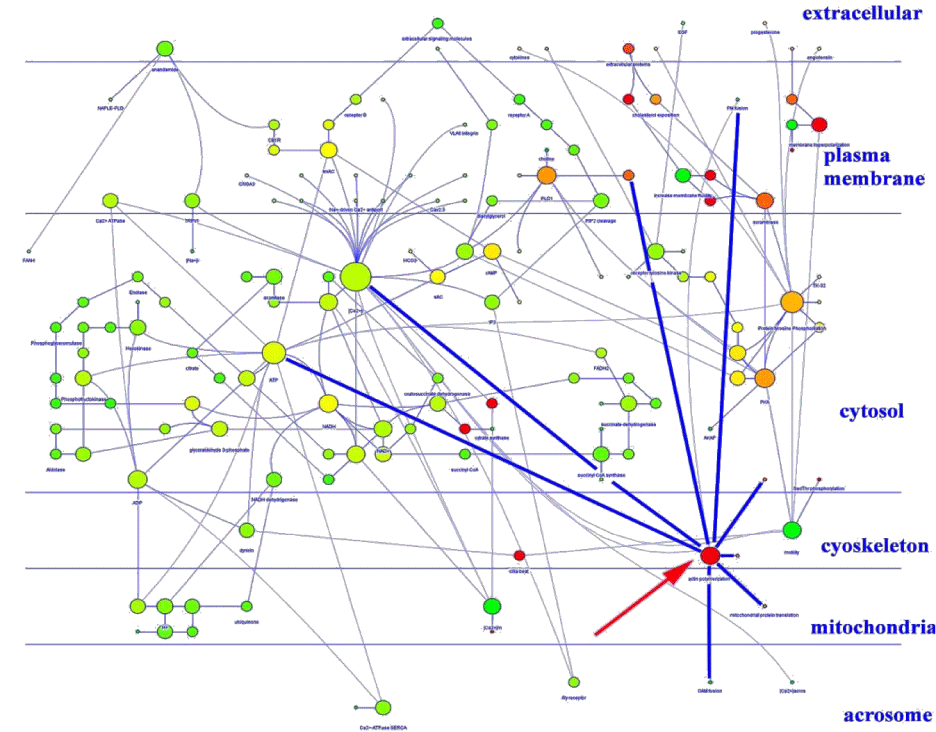Figure 6: Boar spermatozoa capacitation network and with subcellular localization of nodes. The node size is proportional to the connection number and the node color gradient, from red (higher values) to green (lower values) is dependent from the closeness centrality. This parameter is computed as: Cc(n) = 1/avg(L(n,m)), were L (n,m) is the length of the shortest path between two nodes n and m. The closeness centrality of each node ranges from 0 (red) to 1 (green) and it is a measure of how fast the information spreads from a given node to the other nodes (modified from Bernabò et al. ).